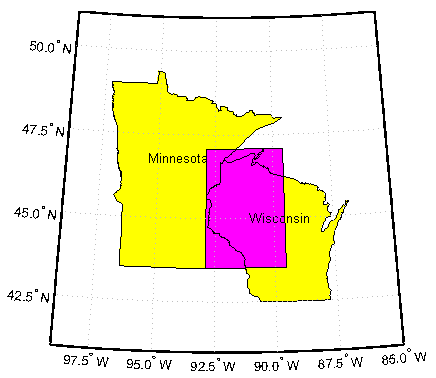## Syntax

```[latlim, lonlim] = intersectgeoquad(latlim1, lonlim1, latlim2, lonlim2) ```

## Description

```[latlim, lonlim] = intersectgeoquad(latlim1, lonlim1, latlim2, lonlim2)``` computes the intersection of the quadrangle defined by the latitude and longitude limits `latlim1` and `lonlim1`, with the quadrangle defined by the latitude and longitude limits `latlim2` and `lonlim2`. `latlim1` and `latlim2` are two-element vectors of the form `[southern-limit northern-limit]`. Likewise, `lonlim1` and `lonlim2` are two-element vectors of the form `[western-limit eastern-limit]`. All input and output angles are in units of degrees. The intersection results are given in the output arrays `latlim` and `lonlim`. Given an arbitrary pair of input quadrangles, there are three possible results:

1. The quadrangles fail to intersect. In this case, both `latlim` and `lonlim` are empty arrays.

2. The intersection consists of a single quadrangle. In this case, `latlim` (like `latlim1` and `latlim2`) is a two-element vector that has the form ```[southern-limit northern-limit]```, where `southern-limit` and `northern-limit` represent scalar values. `lonlim` (like `lonlim1` and `lonlim2`), is a two-element vector that has the form ```[western-limit eastern-limit]```, with a pair of scalar limits.

3. The intersection consists of a pair of quadrangles. This can happen when longitudes wrap around such that the eastern end of one quadrangle overlaps the western end of the other and vice versa. For example, if `lonlim1 = [-90 90]` and ```lonlim2 = [45 -45]```, there are two intervals of overlap: ```[-90 -45]``` and `[45 90]`. These limits are returned in `lonlim` in separate rows, forming a 2-by-2 array. In our example (assuming that the latitude limits overlap), `lonlim` would equal `[-90 -45; 45 90]`. It still has the form ```[western-limit eastern-limit]```, but `western-limit `and `eastern-limit` are 2-by-1 rather than scalar. The two output quadrangles have the same latitude limits, but these are replicated so that `latlim` is also 2-by-2.

To continue the example, if `latlim1 = [0 30]` and ```latlim2 = [20 50]```, `latlim` equals ```[20 30; 20 30]```. The form is still `[southern-limit northern-limit]`, but in this case `southern-limit` and `northern-limit` are 2-by-1.

## Examples

### Example 1

```[latlim, lonlim] = intersectgeoquad( ... [-40 -60], [-180 180], [40 60], [-180 180]) latlim = [] lonlim = [] ```

### Example 2

```[latlim, lonlim] = intersectgeoquad( ... [-40 60], [-120 45], [-60 40], [160 -75]) latlim = -40 40 lonlim = -120 -75```

### Example 3

Intersection is a pair of quadrangles:

```[latlim, lonlim] = intersectgeoquad( ... [-30 90],[-10 -170],[-90 30],[170 10]) latlim = -30 30 -30 30 lonlim = -10 10 170 -170```

### Example 4

Inputs and output fully encircle the planet:

```[latlim, lonlim] = intersectgeoquad( ... [-30 90],[-180 180],[-90 30],[0 360]) latlim = -30 30 lonlim = -180 180```

### Example 5

Find and map the intersection of the bounding boxes of adjoining U.S. states:

```usamap({'Minnesota','Wisconsin'}) S = shaperead('usastatehi','UseGeoCoords',true,'Selector',... {@(name) any(strcmp(name,{'Minnesota','Wisconsin'})), 'Name'}); geoshow(S, 'FaceColor', 'y') textm([S.LabelLat], [S.LabelLon], {S.Name},... 'HorizontalAlignment', 'center') latlimMN = S(1).BoundingBox(:,2)' latlimMN = 43.4995 49.3844 lonlimMN = S(1).BoundingBox(:,1)' lonlimMN = -97.2385 -89.5612 latlimWI = S(2).BoundingBox(:,2)' latlimWI = 42.4918 47.0773 lonlimWI = S(2).BoundingBox(:,1)' lonlimWI = -92.8892 -86.8059 [latlim lonlim] = ... intersectgeoquad(latlimMN, lonlimMN, latlimWI, lonlimWI) latlim = 43.4995 47.0773 lonlim = -92.8892 -89.5612 geoshow(latlim([1 2 2 1 1]), lonlim([1 1 2 2 1]), ... 'DisplayType','polygon','FaceColor','m') ```## Tips

`latlim1` and `latlim2` should normally be given in order of increasing numerical value. No error will result if, for example, `latlim1(2) < latlim1(1)`, but the outputs will both be empty arrays.

No such restriction applies to `lonlim1` and `lonlim2`. The first element is always interpreted as the western limit, even if it exceeds the second element (the eastern limit). Furthermore, `intersectgeoquad` correctly handles whatever longitude-wrapping convention may have been applied to `lonlim1` and `lonlim2`.

In terms of output, `intersectgeoquad` wraps `lonlim` such that all elements fall in the closed interval `[-180 180]`. This means that if (one of) the output quadrangle(s) crosses the 180° meridian, its western limit exceeds its eastern limit. The result would be such that

`lonlim(2) < lonlim(1)`
if the intersection comprises a single quadrangle or
`lonlim(k,2) < lonlim(k,1)`
where `k` equals 1 or 2 if the intersection comprises a pair of quadrangles.

If `abs(diff(lonlim1))` or `abs(diff(lonlim2))` equals `360`, its quadrangle is interpreted as a latitudinal zone that fully encircles the planet, bounded only by one parallel on the south and another parallel on the north. If two such quadrangles intersect, `lonlim` is set to `[-180 180]`.

If you want to display geographic quadrangles generated by this function or any other which are more than one or two degrees in extent, they may not follow curved meridians and parallels very well. The degree of departure depends on the extent of the quadrangle, the map projection, and the map scale. In such cases, you can interpolate intermediate vertices along quadrangle edges with the `outlinegeoquad` function.

## Version History

Introduced in R2008a